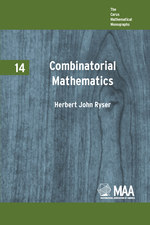Have library access? Log in through your library# Combinatorial Mathematics

HERBERT JOHN RYSER
Volume: 14
Copyright Date: 1963
Edition: 1
Pages: 172
https://www.jstor.org/stable/10.4169/j.ctt5hh8v6

## Table of Contents

1. Front Matter
(pp. i-viii)
2. PREFACE
(pp. ix-x)
Herbert J. Ryser
3. ACKNOWLEDGMENTS
(pp. xi-xii)
4. Table of Contents
(pp. xiii-xvi)
5. CHAPTER 1 FUNDAMENTALS OF COMBINATORIAL MATHEMATICS
(pp. 1-16)

Combinatorial mathematics, also referred to as combinatorial analysis or combinatorics, is a mathematical discipline that began in ancient times. According to legend the Chinese Emperor Yu (c. 2200 B.C.) observed the magic square

$\left( {\begin{array}{*{20}{c}} 4 & 9 & 2 \\ 3 & 5 & 7 \\ 8 & 1 & 6 \\ \end{array}} \right)$

on the back of a divine tortoise. Permutations had a feeble beginning in China before 1100 B.C., and Rabbi Ben Ezra (c. 1140 A.D.) seems to have known the formula for the number of combinations ofnthings takenrat a time. Much of the earliest work is tied to number mysticism. But during the last few centuries various writers have approached the subject from...

6. CHAPTER 2 THE PRINCIPLE OF INCLUSION AND EXCLUSION
(pp. 17-28)

LetSbe ann-set. Let each$a \in S$be assigned a uniqueweight$W(a)$with$W(a)$an element of a fieldF. No restrictions are placed on the fieldFor on the weight assignments withinF. But frequently the combinatorial problem under consideration suggests some natural weight assignment. In many problems each$a \in S$is assigned a weight$W(a)$equal to the positive integer 1. LetPdenote anN-set ofNproperties

(1.1)${P_1},{P_2},...,{P_N}$

connected with the elements ofSand let

(1.2)$\{ {P_{{i_1}}},{P_{{i_2}}},.,{P_{{i_r}}}\}$

denote anr-subset ofP. Let

(1.3)$W({P_{{i_1}}},{P_{{i_2}}},.,{P_{{i_r}}})$

equal the sum of the weights of...

7. CHAPTER 3 RECURRENCE RELATIONS
(pp. 29-37)

The relationship

(1.1)$\left( \begin{array}{l} n \\ r \\ \end{array} \right) = \left( \begin{array}{l} n - 1 \\ r \\ \end{array} \right) + \left( \begin{array}{l} n - 1 \\ r - 1 \\ \end{array} \right)$

is a simple instance of a recurrence. From (1.1) and the appropriate initial values one may evaluate the binomial coefficients for all nonnegative integersnandr. The evaluation procedure is schematically illustrated by the Pascal triangle. Relationships of many different types and varieties are called recurrences, and there is no need for us to insist on a formal definition of the term. But by and large a recurrence stands for a special type of relationship involving a quantity with integer parameters. This relationship is such that it may be used to evaluate the quantity term...

8. CHAPTER 4 A THEOREM OF RAMSEY
(pp. 38-46)

We devote this section to the description and proof of an important combinatorial theorem that originated in certain investigations in the foundations of mathematics. The theorem is called Ramsey’s theorem after the English logician F. P. Ramsey. The pigeon-hole principle in mathematics asserts that if a set of sufficiently many elements is partitioned into not too many subsets, then at least one of the subsets must contain many of the elements. Ramsey’s theorem may be regarded as a profound generalization of this simple principle. The proof of Ramsey’s theorem makes extensive use of recurrence techniques. The theorem has a wide...

9. CHAPTER 5 SYSTEMS OF DISTINCT REPRESENTATIVES
(pp. 47-60)

LetSbe an arbitrary set and let$P(S)$denote the set of all subsets ofS. Let

(1.1)$D = ({a_1},{a_2},...,{a_m})$

be anm-sample ofSand let

(1.2)$M(S) = ({S_1},{S_2},...,{S_m})$

be anm-sample of$P(S)$. Now suppose that themelements ofDare distinct and suppose that

(1.3)${a_i} \in {S_i}$($(i = 1,2,...,m)$).

Then the element${a_i}$represents the set${S_i}$, and we say that the subsets${S_1},{S_2},...,{S_m}$have asystem of distinct representatives(abbreviated SDR). We callDan SDR for$M(S)$. The definition of SDR requires${a_i} \ne {a_j}$whenever$i \ne j$but${S_i}$and${S_j}$need not be distinct as subsets ofS....

10. CHAPTER 6 MATRICES OF ZEROS AND ONES
(pp. 61-78)

LetAbe a (0, 1)-matrix of sizembyn. Let the sum of rowiofAbe denoted by${r_i}$and let the sum of columnjofAbe denoted by${s_j}$. We call the vector

(1.1)$R = ({r_1},{r_2},...,{r_m})$

therow sum vectorand the vector

(1.2)$S = ({s_1},{s_2},...,{s_n})$

thecolumn sum vectorofA. We sayRismonotoneprovided${r_1}\underline \ge {r_2}\underline \ge ...\underline \ge {r_m}$, and a similar definition holds forS. Ifrdenotes the total number of 1’s inA, then it i clear that

(1.3)$\tau = \sum\limits_{i = 1}^m {{r_i}} = \sum\limits_{j = 1}^n {{s_j}}$

The vectorsRandSdetermine a class

(1.4)$u = u(R,S)$

consisting of...

11. CHAPTER 7 ORTHOGONAL LATIN SQUARES
(pp. 79-95)

Let${A_1} = [{a_{ij}}^{(1)}]$and${A_2} = [{a_{ij}}^{(2)}]$denote twonbynLatin squares based onnelements labeled$1,2,...,n$and let$n\underline \ge 3$. The Latin squares${A_1}$and${A_2}$are calledorthogonalprovided that the${n^2}$2-samples

(1.1)$({a_{ij}}^{(1)},{a_{ij}}^{(2)})$$(i,j = 1,2,...,n)$

are distinct. In other words suppose that one of the Latin squares is superimposed on the other. Then the resulting configuration is annbynarray of ordered pairs of 1, 2, . . . ,n, and the orthogonality requirement on the Latin squares means that all of the entries in this array are distinct. Thenbynarray of...

12. CHAPTER 8 COMBINATORIAL DESIGNS
(pp. 96-130)

In this section we introduce combinatorial configurations that are generalizations of the finite projective planes described in the preceding chapter. LetXbe av-set of elements${x_1},{x_2},...,{x_0}$and let${X_1},{X_2},...,{X_b}$bebdistinct subsets ofX. These subsets are called abalanced incomplete block designprovided they satisfy the following requirements.

(1.1) Each${x_i}$, is ak-subset ofX.

(1.2) Each 2-subset ofXis a subset of exactly λ of the sets${X_1},{X_2},...,{X_b}$

(1.3) The integersv, k,and λ satisfy$0 < \lambda$and$k < v - 1$

Requirements (1.1) and (1.2) are basic to the definition of balanced incomplete block designs,...

13. CHAPTER 9 PERFECT DIFFERENCE SETS
(pp. 131-142)

Let$D = \{ {d_1},{d_2},.,{d_k}\}$be ak-set of integers modulovsuch that every${\rm A} \ne 0$(modv) can be expressed in exactly λ ways in the form

(1.1)${d_i} - {d_j} \equiv a(\bmod v)$,

where${d_i}$and${d_j}$are inD. We suppose further that

(1.2)$0 < \lambda < k < v - 1$.

The inequality (1.2) serves only to exclude certain degenerate configurations. A setDfulfilling these requirements is called aperfect difference setor, for brevity, adifference set.It is easy to verify that

(1.3)$\lambda = \frac{{k(k - 1)}} {{v - 1}}$.

This assertion is also an immediate consequence of the following theorem.

THEOREM 1.1.The perfect difference set D is equivalent to$a(v,k,\lambda )$-configuration with incidence...

14. LIST OF NOTATION
(pp. 143-146)
15. INDEX
(pp. 147-154)